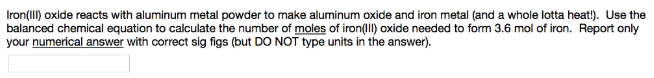# Problem: Iron (III) oxide reacts with aluminum metal powder to make aluminum oxide and iron metal (and a whole lotta heat!). Use the balanced chemical equation to calculate the number of moles of iron(lll) oxide needed to form 3.6 mol of iron. Report only your numerical answer with correct sig figs (but DO NOT type units in the answer).

###### FREE Expert Solution
83% (479 ratings)###### Problem Details

Iron (III) oxide reacts with aluminum metal powder to make aluminum oxide and iron metal (and a whole lotta heat!). Use the balanced chemical equation to calculate the number of moles of iron(lll) oxide needed to form 3.6 mol of iron. Report only your numerical answer with correct sig figs (but DO NOT type units in the answer).